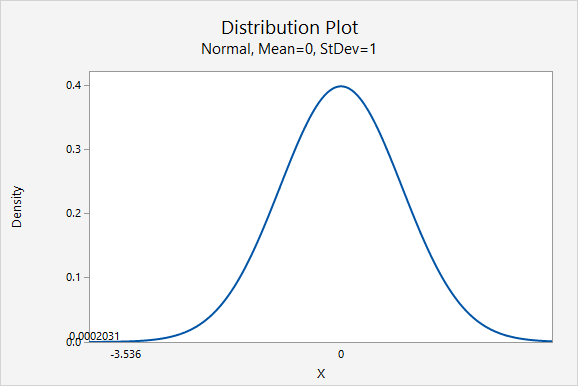# 8.1.2.1.3 - Example: Ice Cream

8.1.2.1.3 - Example: Ice Cream

Research Question: Is the percentage of Creamery customers who prefer chocolate ice cream over vanilla less than 80%?

In a sample of 50 customers 60% preferred chocolate over vanilla.

1. Check assumptions and write hypotheses

$$np_0 = 50(0.80) = 40$$

$$n(1-p_0)=50(1-0.80) = 10$$

Both $$np_0$$ and $$n(1-p_0)$$ are at least 10. We can use the normal approximation method.

This is a left-tailed test because we want to know if the proportion is less than 0.80.

$$H_{0}\colon p=0.80$$
$$H_{a}\colon p<0.80$$

2. Calculate the test statistic
Test statistic: One Group Proportion

$$z=\dfrac{\widehat{p}- p_0 }{\sqrt{\frac{p_0 (1- p_0)}{n}}}$$

$$\widehat{p}$$ = sample proportion
$$p_{0}$$ = hypothesize population proportion
$$n$$ = sample size

$$\widehat{p}=0.60$$, $$p_{0}=0.80$$, $$n=50$$

$$z= \dfrac{\widehat{p}- p_0 }{\sqrt{\frac{p_0 (1- p_0)}{n}}}= \dfrac{0.60-0.80}{\sqrt{\frac{0.80 (1-0.80)}{50}}}=-3.536$$

Our $$z$$ test statistic is -3.536.

3. Determine the p-value

This is a left-tailed test so we need to find the area to the right of our test statistic, $$z=-3.536$$.From the Minitab output above, the p-value is 0.0002031

4. Make a decision

$$p \leq.05$$, therefore our decision is to reject the null hypothesis.

5. State a "real world" conclusion

Yes, there is evidence that the percentage of all Creamery customers who prefer chocolate ice cream over vanilla is less than 80%.

  Link ↥ Has Tooltip/Popover Toggleable Visibility